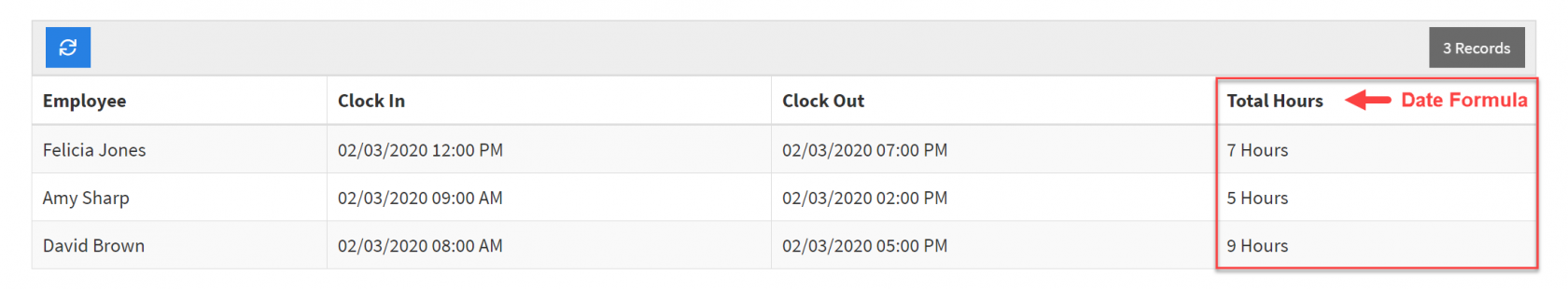# Equation Fields (Category)

## Text Formula

Text Formula fields are basic field types that can combine multiple fields into a single output using a text formula.

Sample Text Formula Field:For instance, if you have a Property Management application with properties and units, you may wish to combine the Property Name field with the Unit Name field to create a new field with the single output of the full Property and Unit name (example: combine "Property A" field with "Unit D5" field to form a new field with the single output of "Property A Unit D5").TIP: Text formulas are similar to "CONCAT" in Excel.

Let's explore how to create a text formula field such as the example above.

### Creating a text formula field

To add a new text formula field to your data table, click on the Equation field type category on the Add Field menu and select the Text Formula field type from the drop-down list.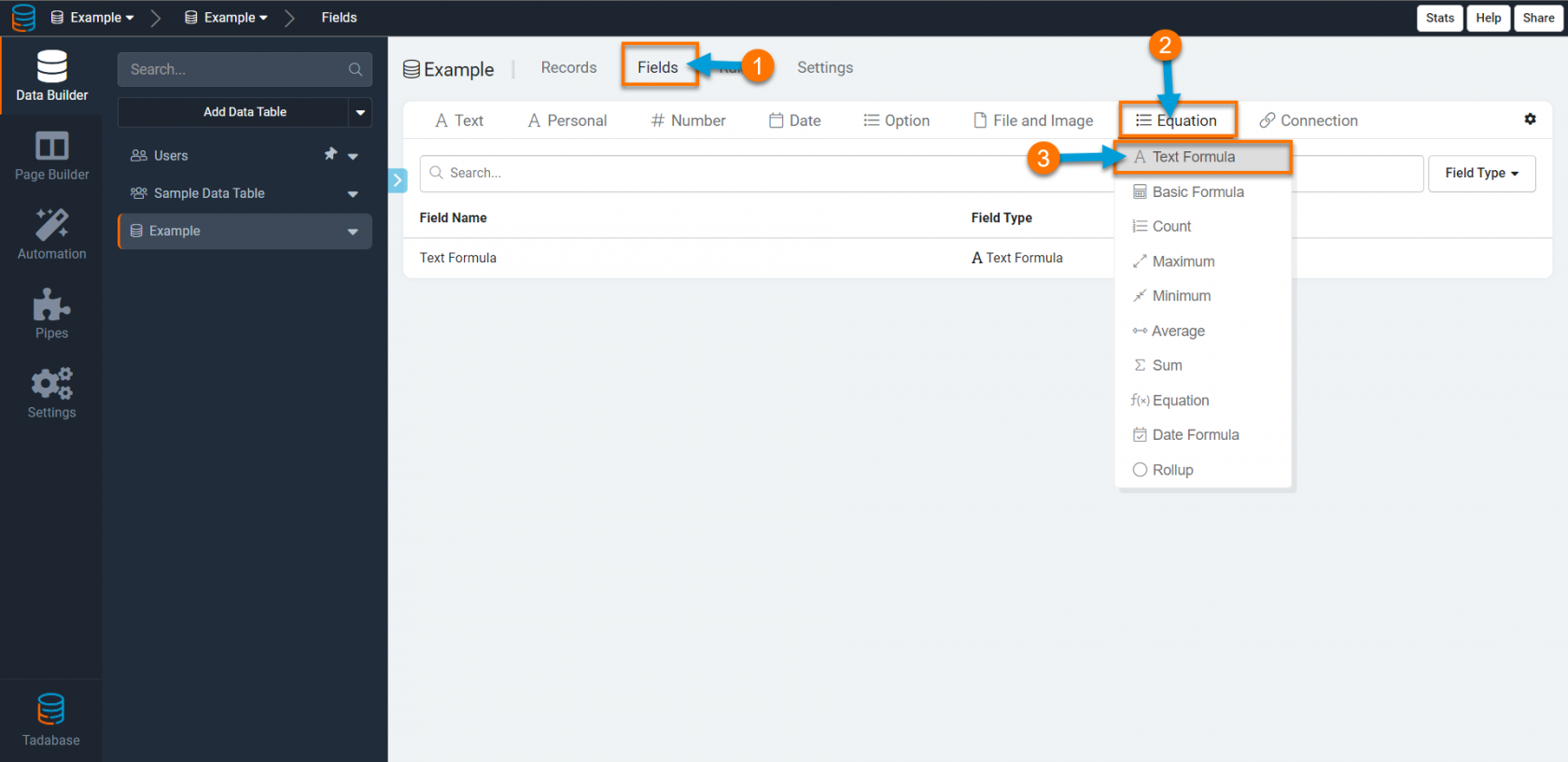When adding a new Text Formula field, there are several settings you can customize such as those highlighted in the image below.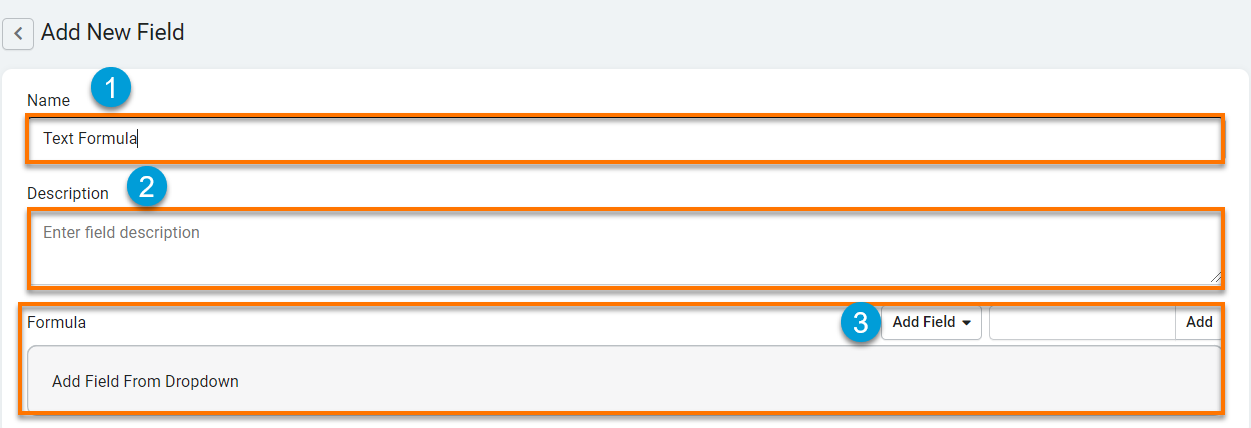1. Name- where you can title your Text Formula field. For instance, "Property and Unit Name" or "Trip Route".

2. Description- where you can optionally provide a brief description of this field for your own reference.

3. Formula- this is where you can define the text formula for this field. The Formula section is divided into three parts: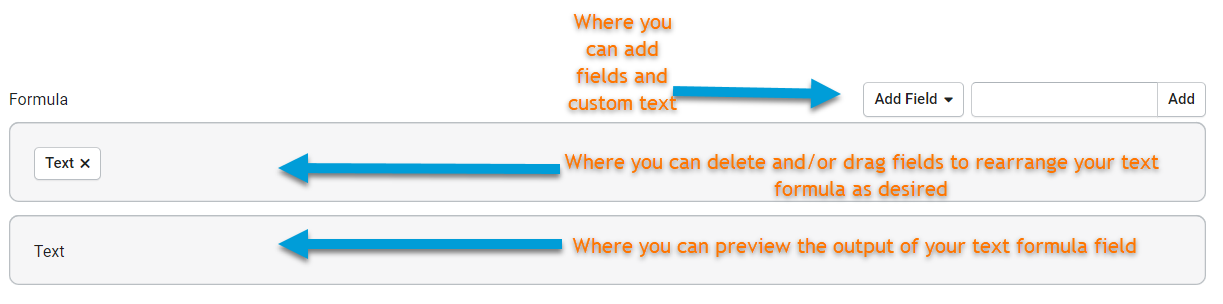Let's discuss how to use the Formula section to create text formulas for your text formula fields.

For instance, in the example listed above we had a Property Name field, a Unit Name field, and we combined these two fields to create a new text formula field titled Property and Unit Name.The GIF below demonstrates the text formula that was used to create this Property and Unit Name text formula field. As you can see in the GIF, the Property Name field is added to the formula, then a custom text of a space character is added, and then the Unit Name field is added to form a single output of "'Property Name' 'space' 'Unit Name'".

Let's walk through another instance of how to create a Text Formula field.

Say you have an Origin field and a Destination field and would like to combine these two fields to create a new field with a single output of your Route (origin to destination). You can accomplish this with a Text Formula field. To create your new Route field, you can create a new Text Formula field, title it "Route", and configure the text formula to combine your Origin and Destination fields into a single output.

As illustrated in the GIF below, you can create your Text Formula field by first clicking on the +Add Field button to add the Origin field. You can then enter the custom text of "'space character' 'to' 'space character'" and clicking on the +Add button to add this custom text. Lastly, you can click on the +Add Field button once again to add the Destination field for a final output of "Origin to Destination". You can then click Save to save your Route Text Formula field.

To test this Text Formula field in action, we can create a simple form in the Page Builder using the Origin and Destination fields.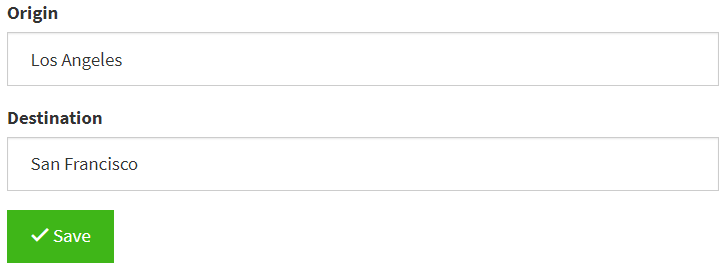When we click Save, we can see our Text Formula field in action within a table component on the live app.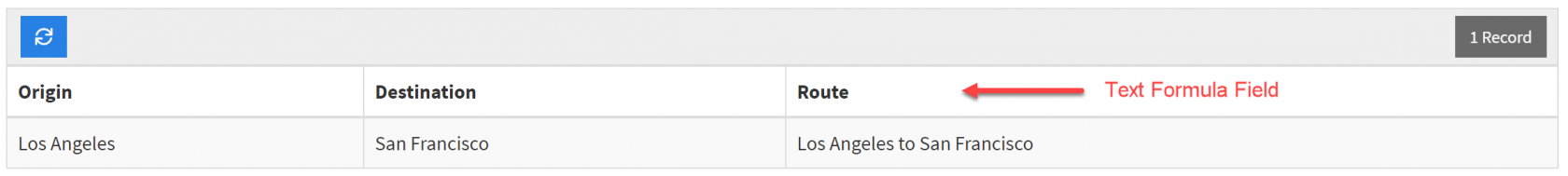## Basic Formula

Basic Formula fields can be used to calculate automatically generated values by combining numeric fields.

For instance, say you have an Orders data table with a Quantity field and a Price field. To calculate the Total Cost of each order, you can create a Basic Formula field with a formula of "Quantity x Price".

Unlike Complex Formulas, Basic Formulas can not be used to calculate values from connected records.

Basic formulas can only be used to calculate numeric fields.

Sample Basic Formula Field: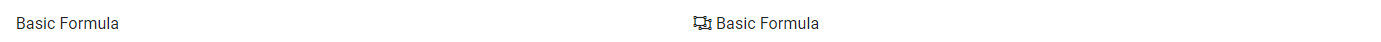### Creating a Basic Formula Field

To add a new Basic Formula field to your data table, click on the Equation field type category on the Add Field menu and select the Basic Formula field type from the drop-down list.When creating a Basic Formula field, there are several settings you can customize such as those highlighted in the image below.1. Name- this is where you can name your Basic Formula field. For instance, "Order Subtotal" or "Remaining Vacation Days".

2. Description- this is where you can optionally provide a brief description of your Basic Formula field for your own reference.

3. Formula- this is where you can create your Basic Formula by combining numeric fields to create a new, automatically calculated field value.

Let's first define the remaining Basic Formula settings of Currency Type, Format, and Decimal Places and then we'll return to this Formula section to demonstrate how to use this section to create a basic formula with numeric fields.

4. Currency Type- this is where you can choose a currency type to display within your Basic Formula field values. At default, the currency type for Basic Formula fields is set to No Currency. However, if you are creating a basic formula to calculate a currency value you may wish to change this setting to a currency type of your choice, such as "\$- USD" or "€- EURO".

5. Format- this is where you can define the location of the currency symbol as it will display within your basic formula currency values. At default, the currency symbol format is set to Prefix, which means that the currency symbol will be displayed before the currency value at default.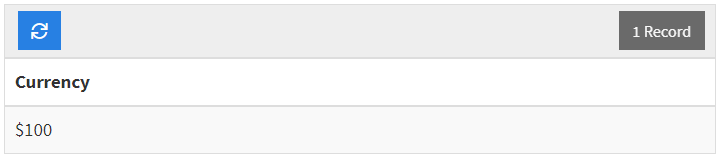You can change the format of your currency symbol location to either None (will not show currency symbol) or Suffix (currency symbol will appear after the currency value).

6. Thousands Delimiter- this is where you can define which character you wish to use as a thousands delimiter to separate groups of thousands when viewing your basic formula values. At default, the thousands delimiter setting for basic formula fields is set to None, meaning your currency values will display with no thousands delimiter at default and will display as such: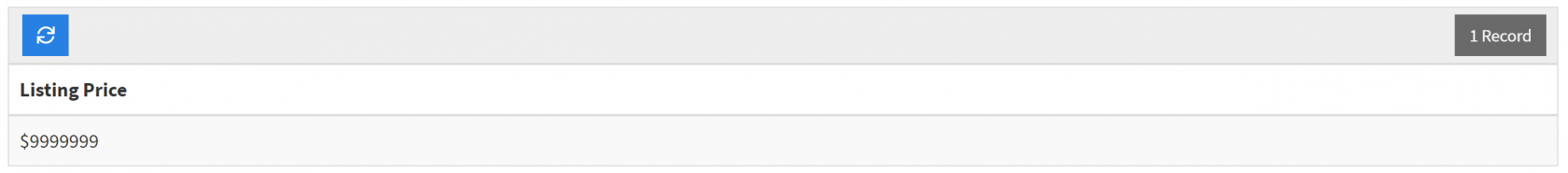If you wish to change your thousands delimiter setting and choose a delimiter character, you can choose either a commaperiod, or space character to separate groups of thousands within your currency values.

For instance, this is how your currency values will display if you change your thousands delimiter setting to Comma: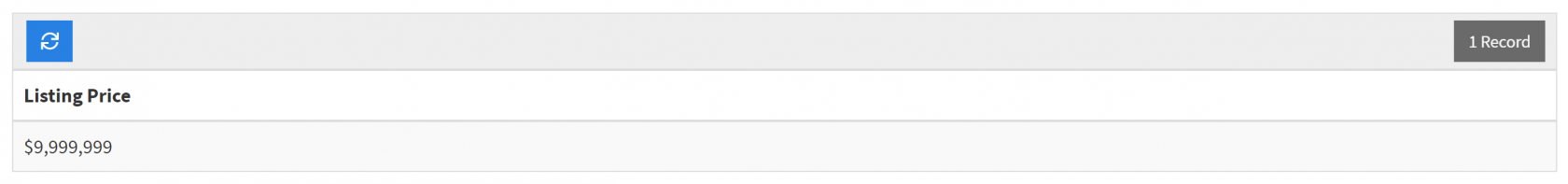7. Decimal Places- this is where you can define how many digits you wish to display after the decimal within your basic formula values. If None or 0 is selected, values will display as whole digits. At default, the decimal place setting for basic formula fields is set to 0 and values will be displayed and stored as whole digits

#### Creating a Basic Formula

Now that we've defined all the settings that you can customize when creating a new Basic Formula field, let's return to the Formula setting to demonstrate how to create a basic formula.

The Formula section of the Basic Formula field can be broken down into three key components as follows: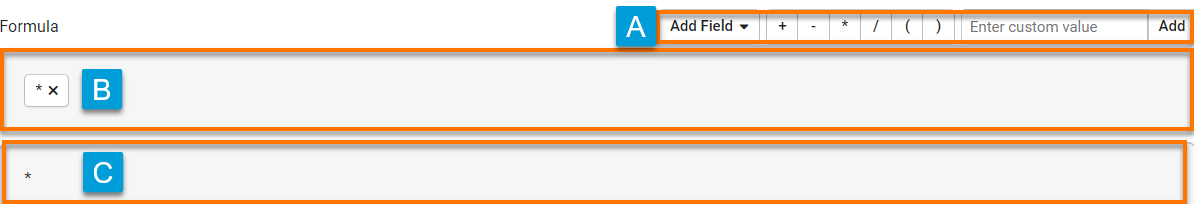A. This is where you can add numeric fields and custom numeric values to create your basic formula.

B. This is where you can delete and/or drag and drop fields that have been added to your formula to arrange your desired field order.

C. This is where you can preview the output of your formula as it will display within your components.

##### An example: Creating a Basic Formula field to calculate employees' remaining vacation days

For instance, if you have an Employee Management application and you would like to generate an automatically calculated value of how many remaining vacation days is available for each employee, you can accomplish this with a Basic Formula field.

To create a Basic Formula field, you must first have at least two numeric fields to work with. In this example, imagine you have two fields-"Starting Vacation Days" and "Used Vacation Days"- and you would now like to create a third automatically generated field to calculate employees' remaining available vacation days. To do this, you would create a Basic Formula field with the formula of "Starting Vacation Days" "-" "Used Vacation Days" to calculate a new numeric field value of "Remaining Vacation Days".

To create this basic formula, select the Basic Formula field type from the Equation field type category.Name your Basic Formula field "Remaining Vacation Days" and click on the +Add Field button to select your first field from the drop-down menu.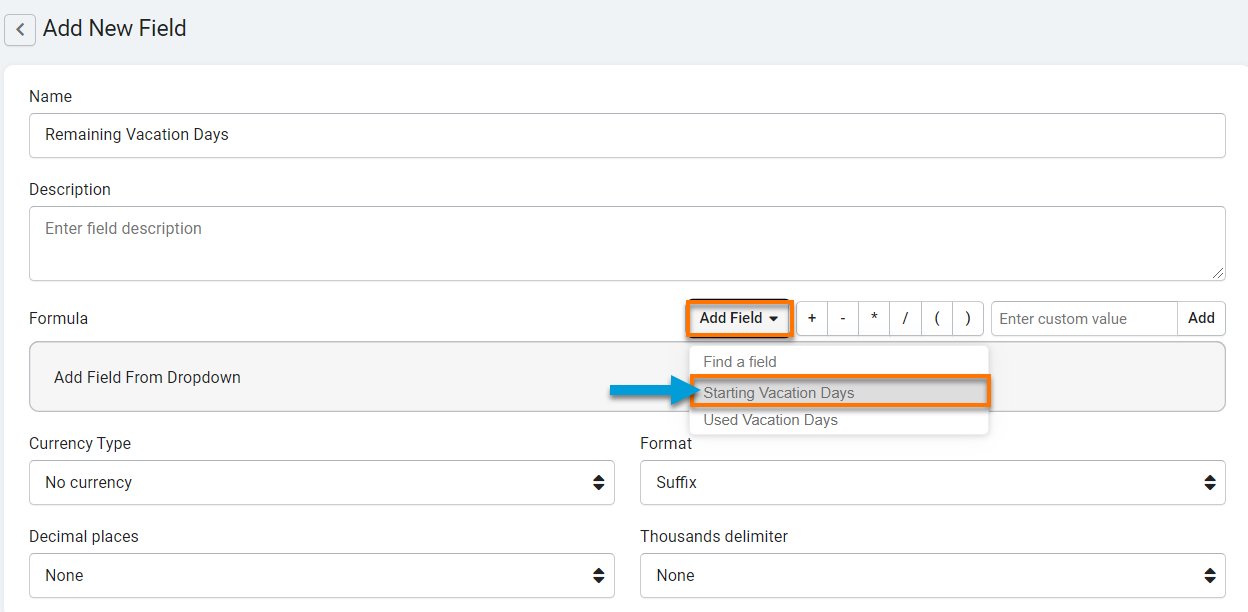Select the "Starting Vacation Days" field to add this field to your formula.You can then select the "-" function to add this function to your formula.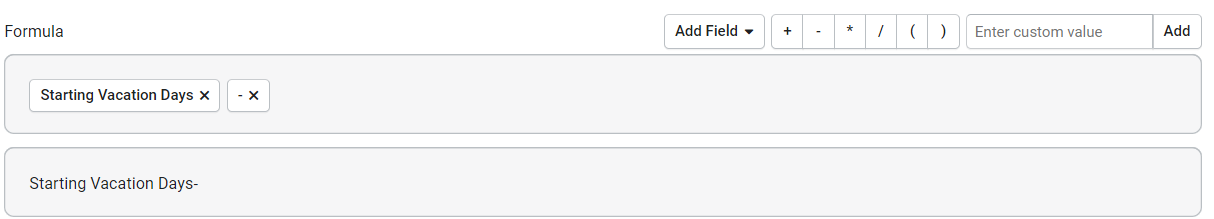Then click on the +Add Field button again to add the "Used Vacation Days" field to your formula. Now that your formula is complete, click Save to save this new Basic Formula field.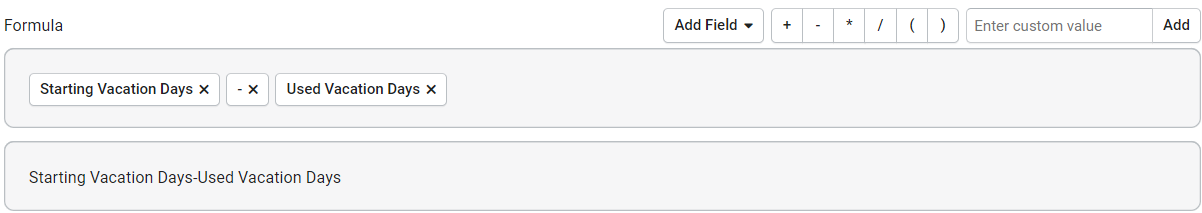You can test your new Basic Formula in action by creating a simple form in the Page Builder using your Starting Vacation Days and Used Vacation Days fields. On the live app, enter values within the form and click Save to save the form.By creating a simple table component within the Page Builder and previewing it on the live app, you can view your basic formula in action. As you can see, your Basic Formula field, titled "Remaining Vacation Days" displays the automatically calculated value of the result of your Basic Formula of Starting Vacation Days - Used Vacation Days.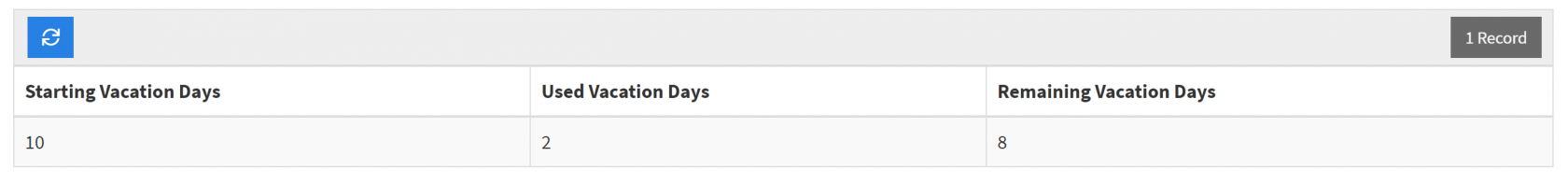### Nesting Basic Formulas

By default, existing basic formulas can not be nested or added inside another basic formula. However, if you need to reference an existing basic formula within your new Basic Formula field, you can accomplish this by recreating your existing formula within parentheses of your new Basic Formula field.

For instance, say you have an existing basic formula of Quantity x Price to calculate the Sub Total of an order.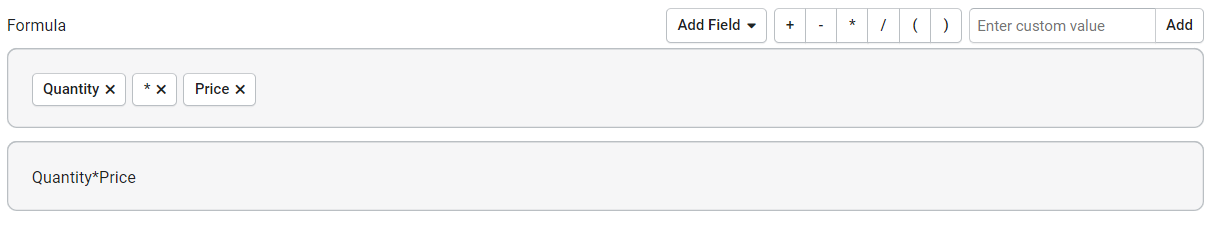If you now wanted to create a new Basic Formula field to calculate the Total with Shipping for each order, you would not be able to nest your existing Sub Total Basic Formula field within your new Total with Shipping formula. What you could do, however, to accomplish the same goal is recreate your Sub Total formula within parentheses of your Total with Shipping formula. Simply recreate your Sub Total formula of Quantity x Price within parentheses and add \$5 shipping to each order by adding the + function and a custom numeric value of 5.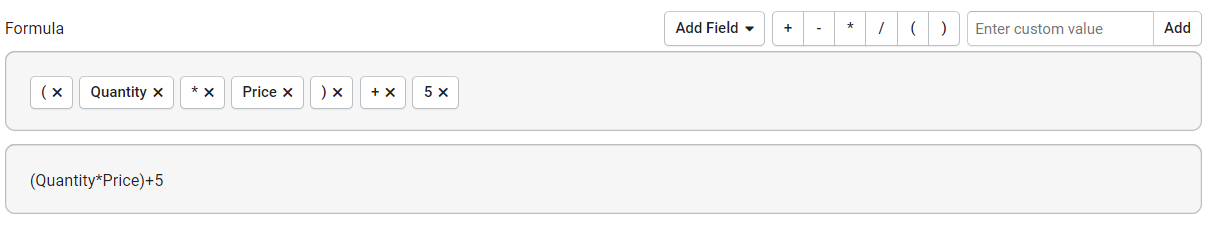You have now created a new Basic Formula field referencing an existing formula field.

## Count

Count fields are complex formula fields that are designed to count records of a connected data table.

Unlike Basic Formulas, Complex Formulas such as Count fields work with connected records.

For instance, say you have an Employees data table, a Tasks data table, and a connection field joining these two data tables which assigns each task to an employee. With a Count field, you can create a complex formula to count the Total Tasks assigned to each employee at any given time.

Sample Count Field: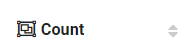### Creating a count field

To add a new Count Field to your data table, click on the Number field type category on the Add Field menu and select the count field type from the drop-down list.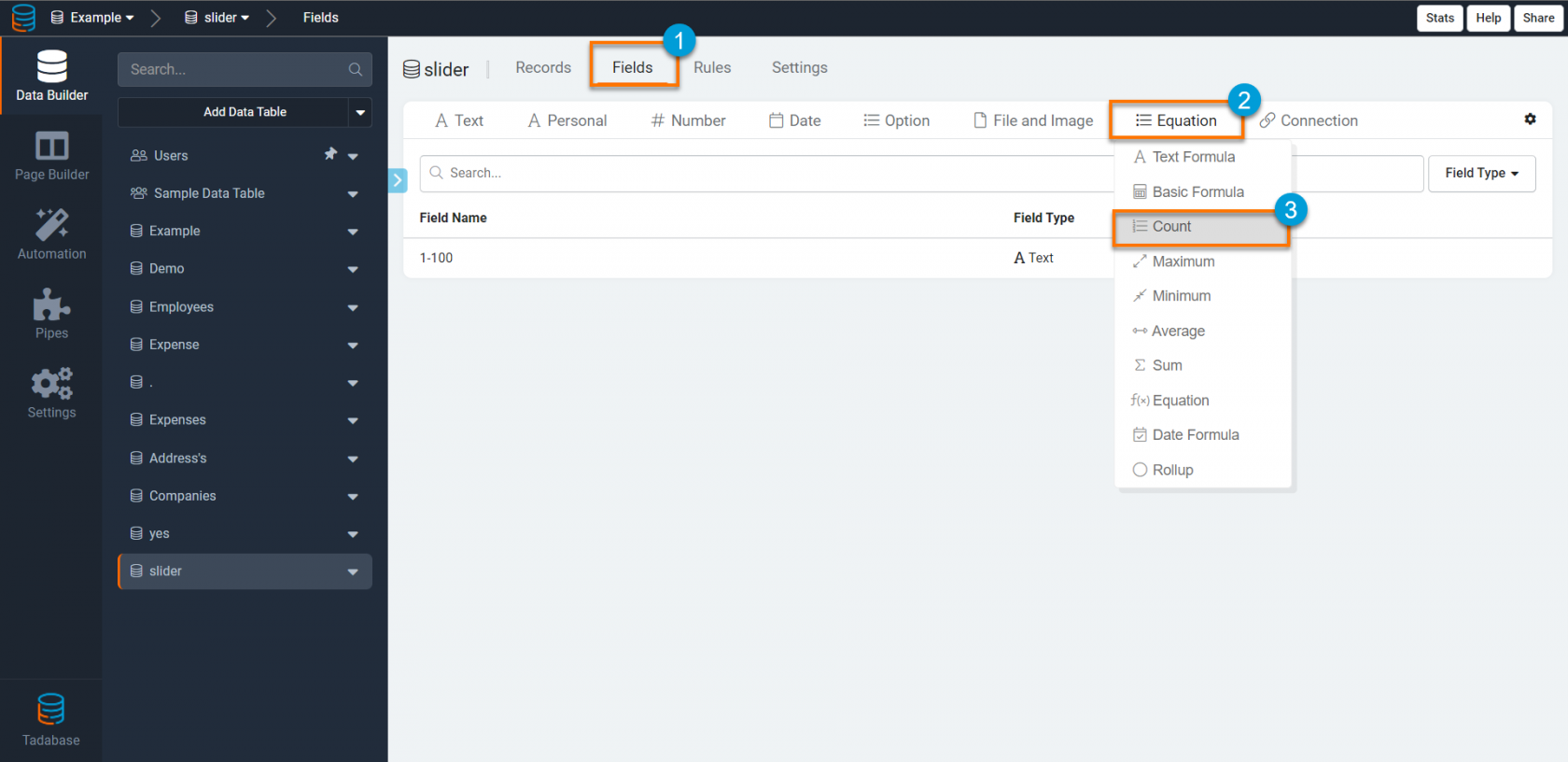When creating a Count field, there are several settings you can customize such as those highlighted in the image below.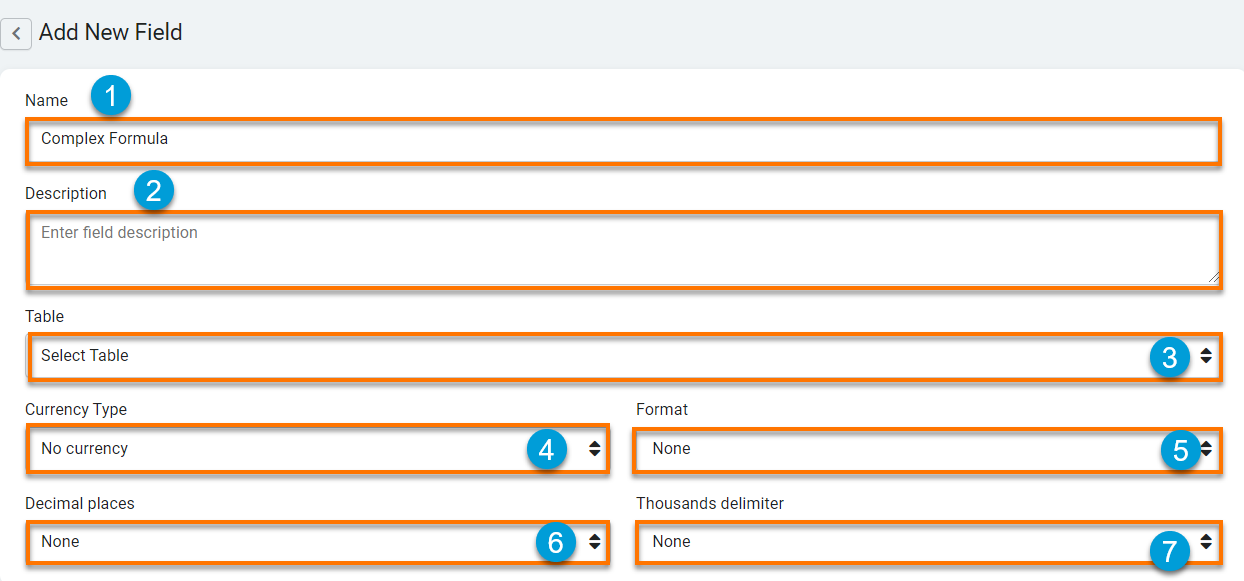1. Name- this is where you can name your Count field. For instance, "Total Jobs" or "Assigned Projects".

2. Description- this is where you can optionally provide a brief description of this field for your own reference.

3. Table- this is where you can select the data table from which you would like to count records.

4. Currency Type- this is where you can choose a currency type to display within your Count field. At default, the currency type for Count fields is set to No Currency. However, if you are creating a Count field to calculate a currency value you may wish to change this setting to a currency type of your choice, such as "\$- USD" or "€- EURO".

5. Format- this is where you can define the location of the currency symbol as it will display within your Count field values. At default, the currency symbol format for Count fields is set to None, which means that no currency symbol will be displayed at default.

If desired, you can change the location formatting of the currency symbol to display either before or after your currency values. For instance, you can change the currency symbol formatting to Prefix which will display the currency symbol before your currency values as illustrated in the image below.6. Thousand Delimiter- this is where you can define which character you wish to use as a thousands delimiter to separate groups of thousands when viewing your currency values. At default, the thousands delimiter setting for Count fields is set to None, meaning your currency values will display with no thousands delimiter at default and will display as such:If you wish to change your thousands delimiter setting and choose a delimiter character, you can choose either a commaperiod, or space character to separate groups of thousands within your currency values.

For instance, this is how your currency values will display if you change your thousands delimiter setting to Comma:7. Decimal Places- this is where you can define how many digits you wish to appear after the decimal within your count field values. At default, the decimal place setting for Count fields is set to None, meaning count field values will display and be stored as whole digits at default.

#### An example: Creating a Count field to count the total jobs for each customer.

For instance, say you have a Customers data table, a Jobs data table, and a connection field joining these two data tables which assigns each job to a customer. With a Count field, you can create a complex formula to count the Total Jobs belonging to each customer at any given time.

To create this Total Jobs Count field, select the Count field type from the Numbers field type category on the Add Field menu within your Customers data table.Name your Count field "Total Jobs" and configure your formula to Count Records from the Jobs data table (where each job is assigned to a customer). Click Save to save your new Total Jobs Count field.You have just added a Total Jobs Count field to your Customers data table that will count the total jobs for each customer. You can view the results of your count field in action via the GIF below where you can see the Total Jobs field for Customer John Simonds dynamically changing each time a new job is assigned to this customer.You can further customize your Total Jobs Count field by adding specific data conditions. For instance, you can add a condition to only include completed jobs within the Total Jobs count.

To do this, click on the Add Data Conditions button within your Total Jobs Count field and create a condition to only count jobs where the "Customer" "is" "Completed". Click save to implement this data condition within your count field.## Maximum

Maximum fields are complex formula fields designed to calculate the maximum value from records of a connected data table.

Unlike Basic Formulas, Complex Formulas such as Maximum fields work with connected records.

For instance, say you have a Students data table, a Grades data table, and a connection field joining these two data tables which assigns each grade to a student. With a Maximum field, you can create a complex formula to calculate each student's Highest Grade at any given time.

Maximum fields can only be used to calculate the maximum value of numeric field values.

Sample Maximum Field:### Creating a Maximum Field

To add a new Maximum field to your data table, click on the Equation field type category on the Add Field menu and select the Maximum field type from the drop-down list.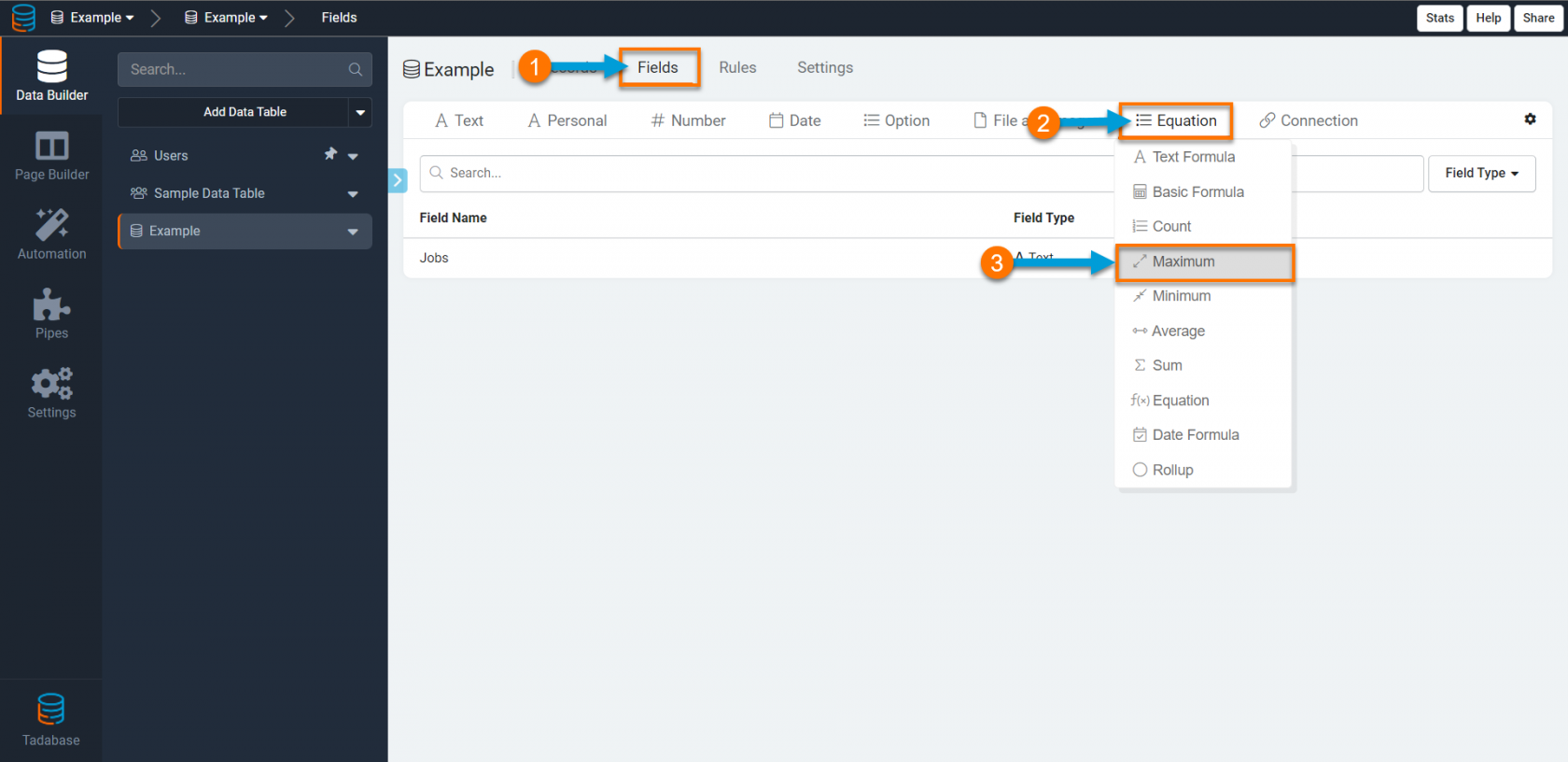When creating a Maximum field, there are several settings you can customize such as those highlighted in the image below.1. Name- this is where you can name your Maximum field. For instance, "Highest Grade" or "Biggest Expense".

2. Description- this is where you can optionally provide a brief description of this field for your own reference.

3. Table- this is where you can select the data table from which you would like to calculate the maximum value.

4. Value Field- this is where you can select the field with which you would like to calculate the maximum value. For instance, if you would like to calculate the highest grade for each student, you would use the Grade field within the Grades data table to calculate this value.

5. Add Data Conditions- this is where you can refine your maximum complex formula by adding specific data conditions. For instance, if you would like to calculate the highest grade for each student within the Fall Semester, you can add a data condition to only calculate the highest grade when the "Semester" "is" "Fall".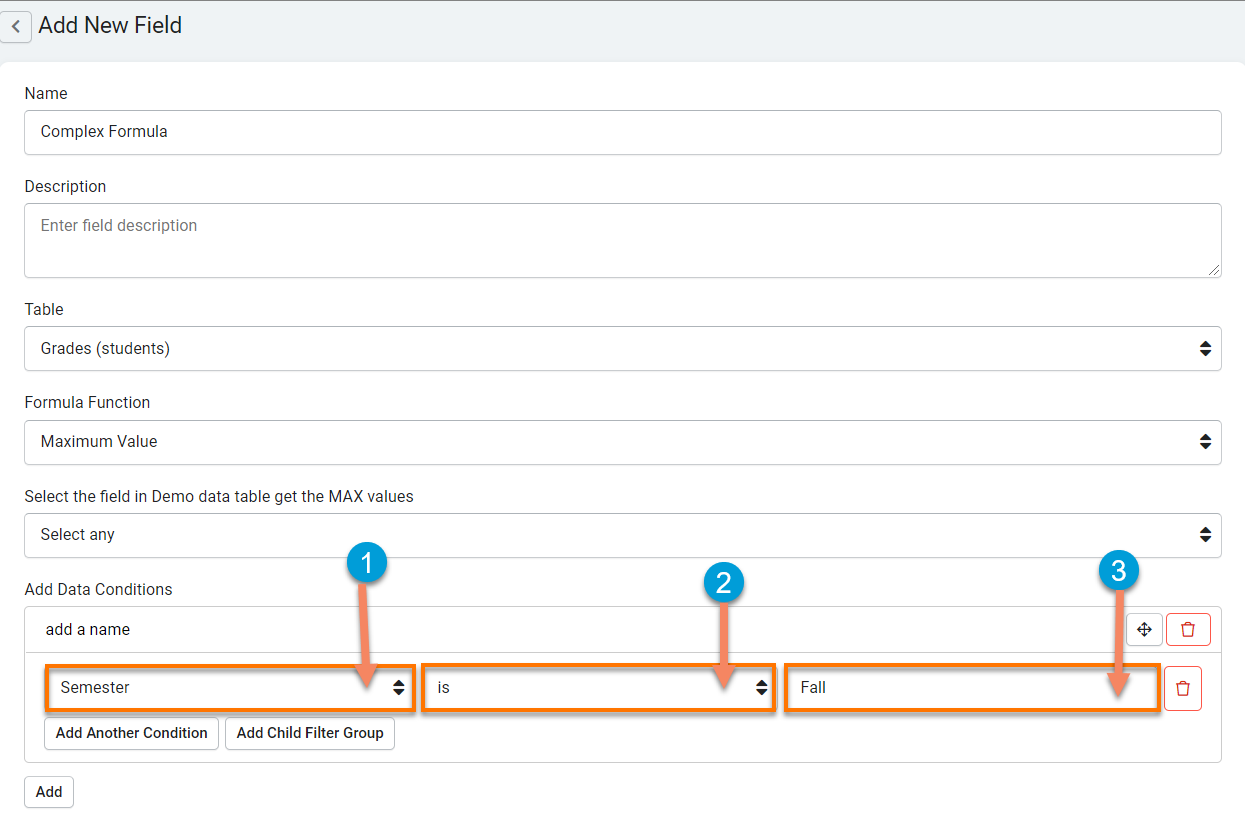6. Currency Type- this is where you can choose a currency type to display within your Maximum field. At default, the currency type for maximum fields is set to No Currency. However, if you are creating a Maximum field to calculate a currency value you may wish to change this setting to a currency type of your choice, such as "\$- USD" or "€- EURO".

7. Format-this is where you can define the location of the currency symbol as it will display within your Maximum field values. At default, the currency symbol format for Maximum fields is set to None, which means that no currency symbol will be displayed at default.

If desired, you can change the location formatting of the currency symbol to display either before or after your currency values. For instance, you can change the currency symbol formatting to Prefix which will display the currency symbol before your currency values as illustrated in the image below.8. Thousand Delimiter- this is where you can define which character you wish to use as a thousands delimiter to separate groups of thousands when viewing your currency values. At default, the thousands delimiter setting for Maximum fields is set to None, meaning your currency values will display with no thousands delimiter at default and will display as such:If you wish to change your thousands delimiter setting and choose a delimiter character, you can choose either a commaperiod, or space character to separate groups of thousands within your currency values.

For instance, this is how your currency values will display if you change your thousands delimiter setting to Comma:9. Decimal Places- this is where you can define how many digits you wish to appear after the decimal within your Maximum field values. At default, the decimal place setting for Maximum fields is set to None, meaning Maximum field values will display and be stored as whole digits at default.

#### An example: Creating a Maximum field to calculate each student's highest grade.

For instance, say you have a Students data table, a Grades data table, and a connection field joining these two data tables which assigns each grade to a student. With a Maximum field, you can create a complex formula to calculate each student's Highest Grade at any given time.

To create this maximum field, click on the Maximum field type on the Equation field type category on the Add Field menu within the Students data table.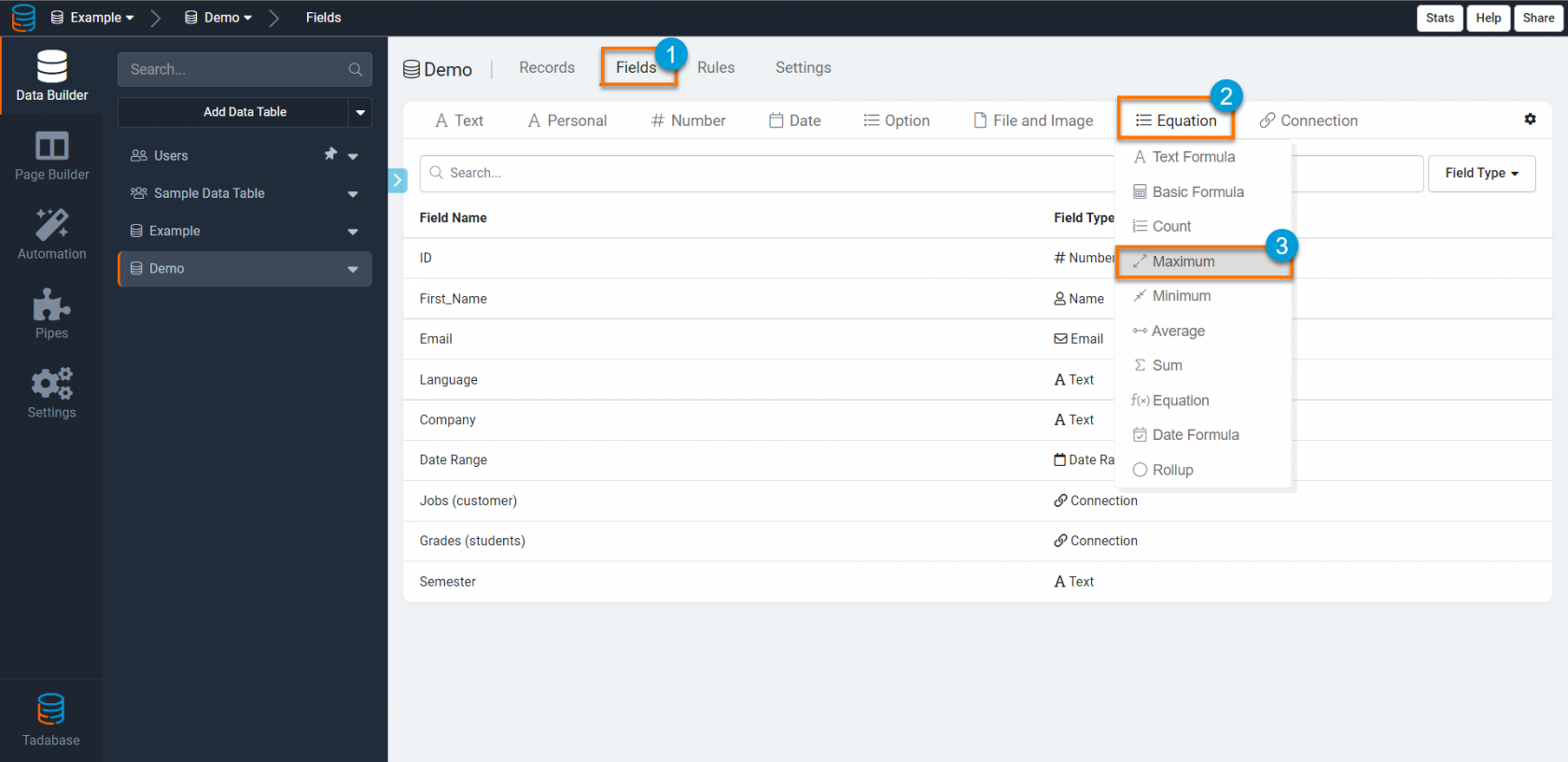Name your Maximum field "Highest Grade" and configure your formula to calculate the maximum value from records within the Grades data table (where each grade is assigned to a student) using the Grade field. Click Save to save your new Highest Grade Maximum field.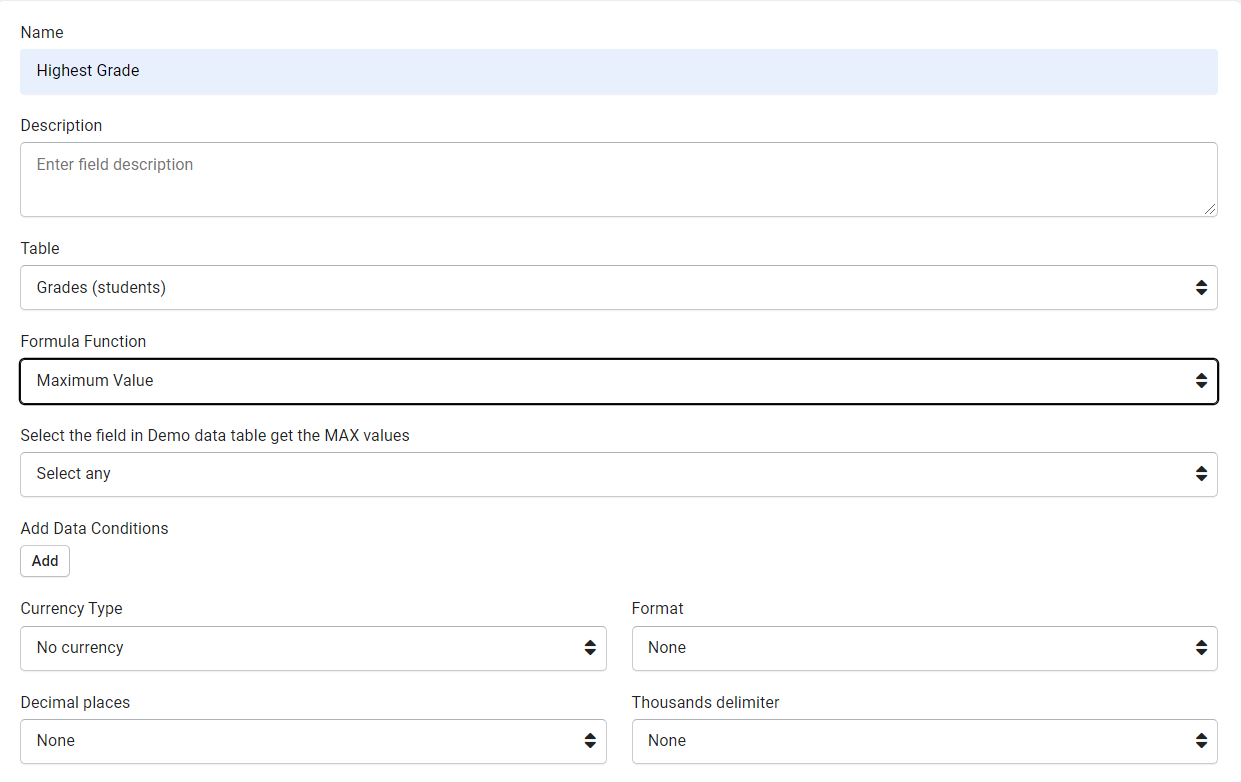You have just added a Highest Grade Maximum field to your Students data table that will calculate each student's highest grade. You can view the results of your Maximum field in action as it would display on your live application via the image below.## Minimum

Minimum fields are complex formula fields designed to calculate the minimum value from records of a connected data table.

Unlike Basic Formulas, Complex Formulas such as Minimum fields work with connected records.

For instance, say you have a Students data table, a Grades data table, and a connection field joining these two data tables which assigns each grade to a student. With a Minimum field, you can create a complex formula to calculate each student's Lowest Grade at any given time.

Minimum fields can only be used to calculate the minimum value of numeric field values.

Sample Minimum Field:### Creating a Minimum Field

To add a new Minimum field to your data table, click on the Number field type category on the Add Field menu and select the Minimum field type from the drop-down list.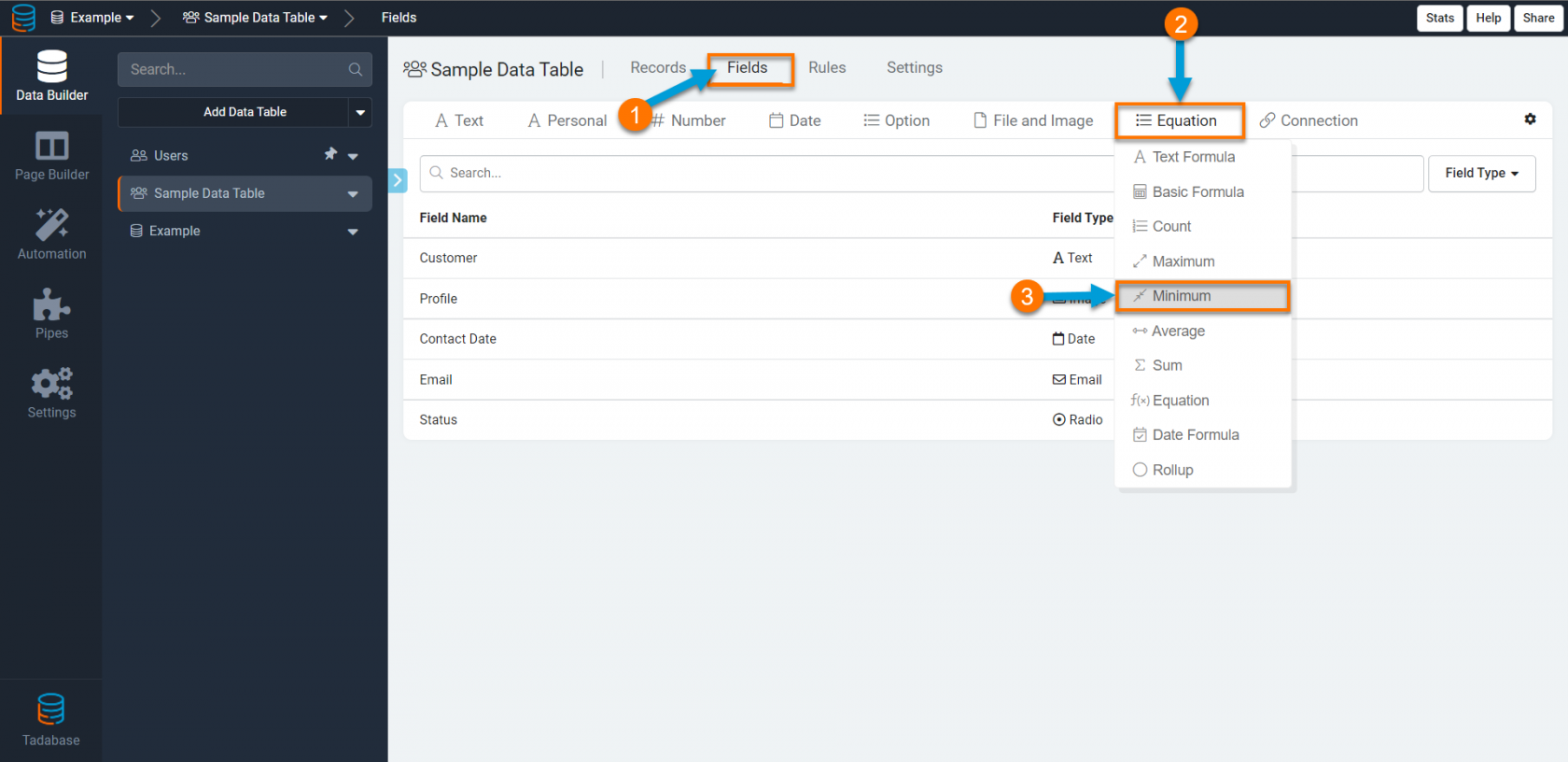When creating a Minimum field, there are several settings you can customize such as those highlighted in the image below.1. Name- this is where you can name your Minimum field. For instance, "Lowest Grade" or "Smallest Expense".

2. Description- this is where you can optionally provide a brief description of this field for your own reference.

3. Formula Function- this is where you can select the function you would like this complex formula to accomplish. As this is a Minimum complex formula field, the default function is set to "Minimum Value".

4. Table- this is where you can select the data table from which you would like to calculate the minimum value.

5. Value Field- this is where you can select the field with which you would like to calculate the minimum value. For instance, if you would like to calculate the lowest grade for each student, you would use the Grade field within the Grades data table to calculate this value.

6. Add Data Conditions- this is where you can refine your minimum complex formula by adding specific data conditions. For instance, if you would like to calculate the lowest grade for each student within the Fall Semester, you can add a data condition to only calculate the lowest grade when the "Semester" "is" "Fall".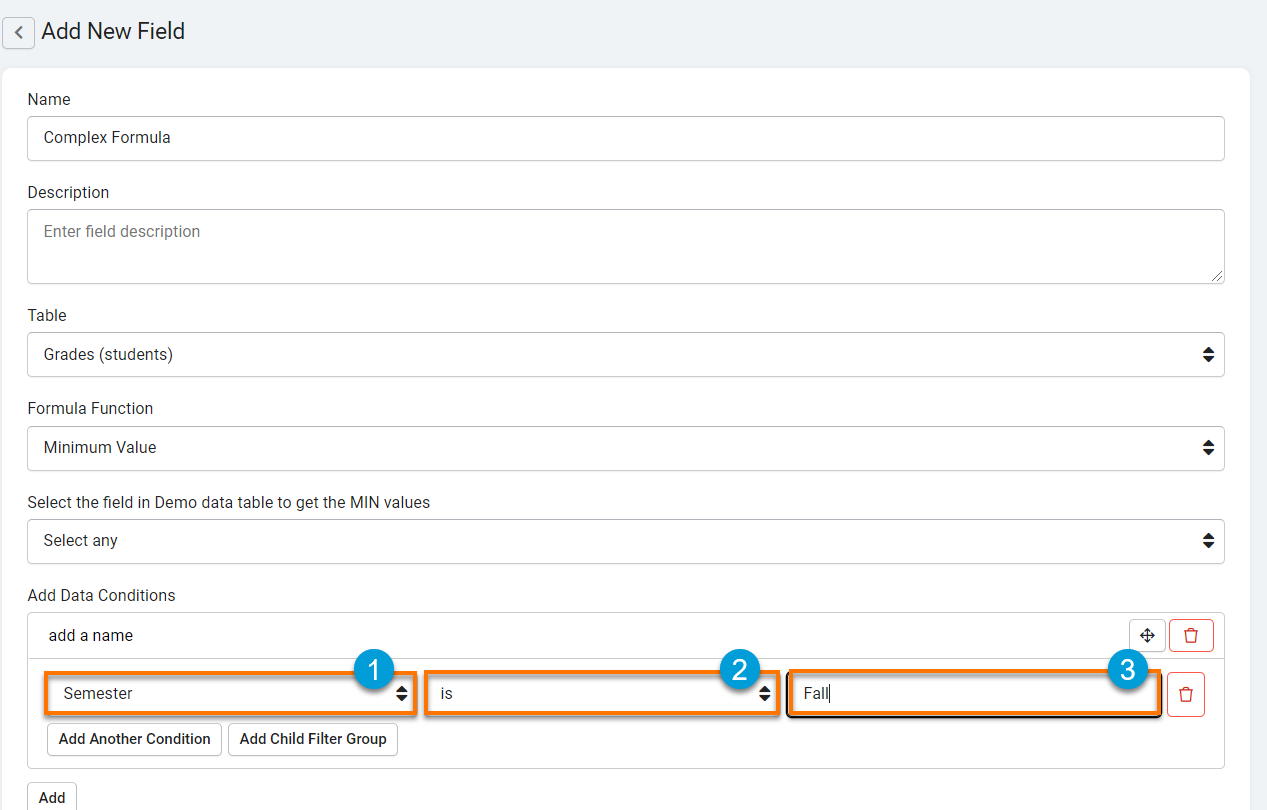7. Currency Type- this is where you can choose a currency type to display within your Minimum field. At default, the currency type for minimum fields is set to No Currency. However, if you are creating a Minimum field to calculate a currency value you may wish to change this setting to a currency type of your choice, such as "\$- USD" or "€- EURO".

8. Format-this is where you can define the location of the currency symbol as it will display within your Minimum field values. At default, the currency symbol format for Minimum fields is set to None, which means that no currency symbol will be displayed at default.

If desired, you can change the location formatting of the currency symbol to display either before or after your currency values. For instance, you can change the currency symbol formatting to Prefix which will display the currency symbol before your currency values as illustrated in the image below.9. Thousand Delimiter- this is where you can define which character you wish to use as a thousands delimiter to separate groups of thousands when viewing your currency values. At default, the thousands delimiter setting for minimum fields is set to None, meaning your currency values will display with no thousands delimiter at default and will display as such:If you wish to change your thousands delimiter setting and choose a delimiter character, you can choose either a commaperiod, or space character to separate groups of thousands within your currency values.

For instance, this is how your currency values will display if you change your thousands delimiter setting to Comma:10. Decimal Places- this is where you can define how many digits you wish to appear after the decimal within your minimum field values. At default, the decimal place setting for Minimum fields is set to None, meaning Minimum field values will display and be stored as whole digits at default.

#### An example: Creating a Minimum field to calculate each student's lowest grade.

For instance, say you have a Students data table, a Grades data table, and a connection field joining these two data tables which assigns each grade to a student. With a Minimum field, you can create a complex formula to calculate each student's Lowest Grade at any given time.

To create this minimum field, click on the Minimum field type on the Number field type category on the Add Field menu within the Students data table.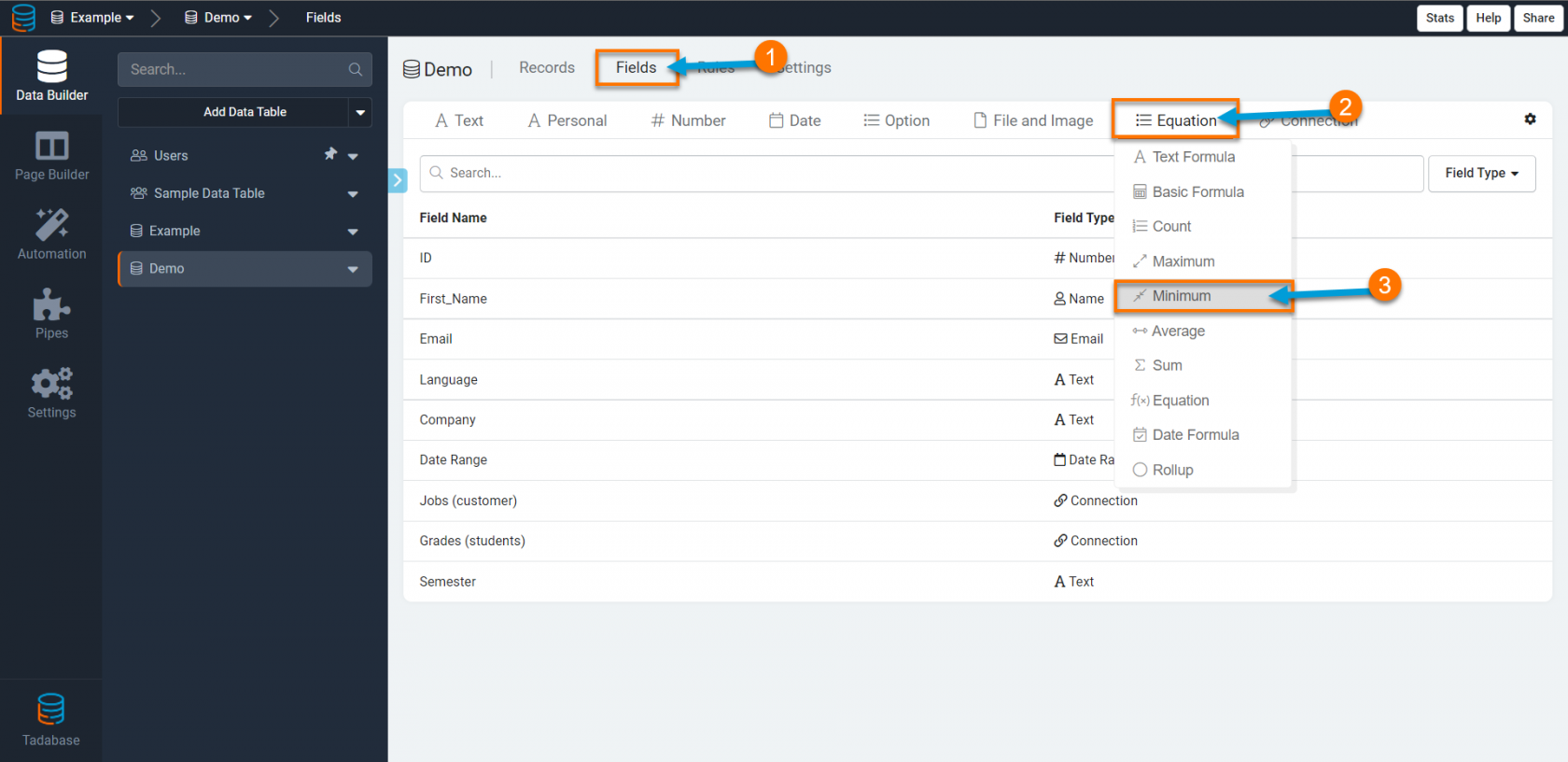Name your Minimum field "Lowest Grade" and configure your formula to calculate the minimum value from records within the Grades data table (where each grade is assigned to a student) using the Grade field. Click Save to save your new Lowest Grade Minimum field.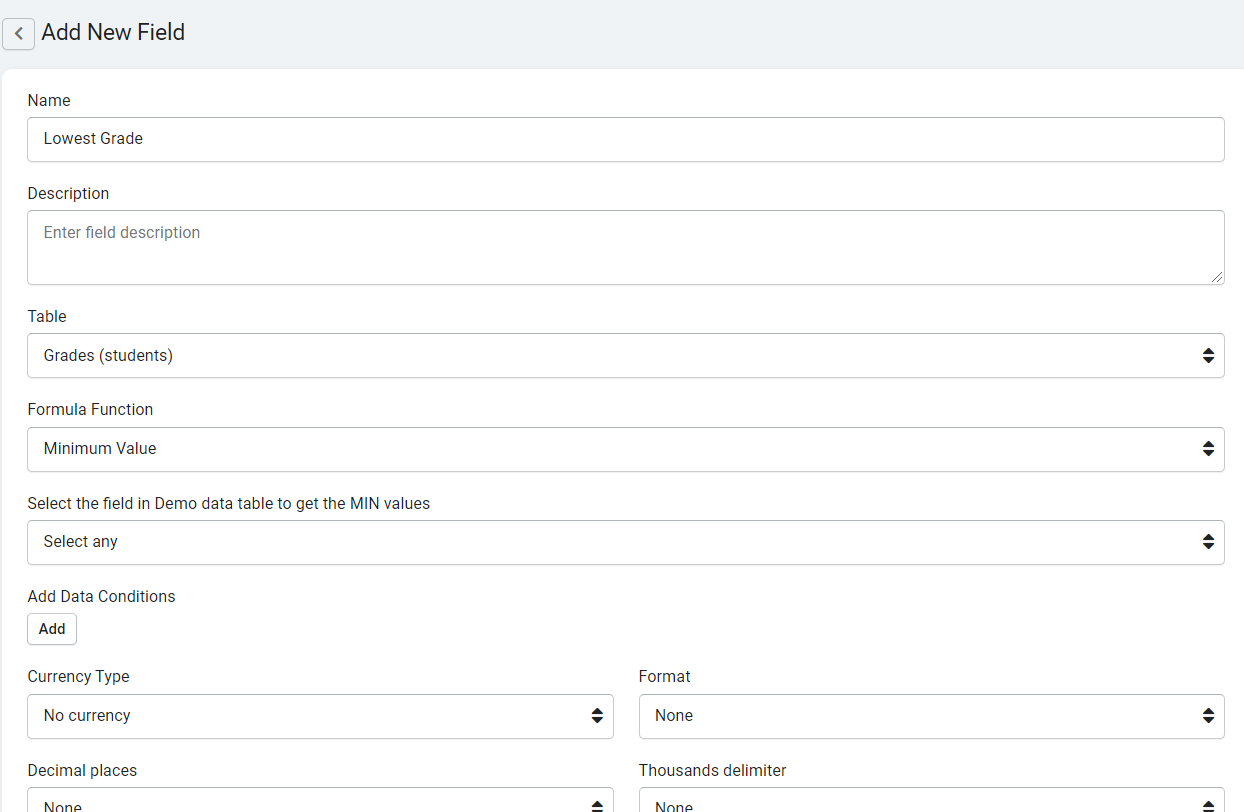You have just added a Lowest Grade Minimum field to your Students data table that will calculate each student's lowest grade. You can view the results of your Minimum field in action as it would display on your live application via the image below.## Average

Average fields are complex formula fields designed to calculate the average value from records of a connected data table.

Unlike Basic Formulas, Complex Formulas such as Average fields work with connected records.

For instance, say you have a Students data table, a Grades data table, and a connection field joining these two data tables which assigns each grade to a student. With an Average field, you can create a complex formula to calculate each student's Average Grade at any given time.

Average fields can only be used to calculate the average value of numeric field values.

Sample Average Field:### Creating an Average Field

To add a new Average field to your data table, click on the Number field type category on the Add Field menu and select the Average field type from the drop-down list.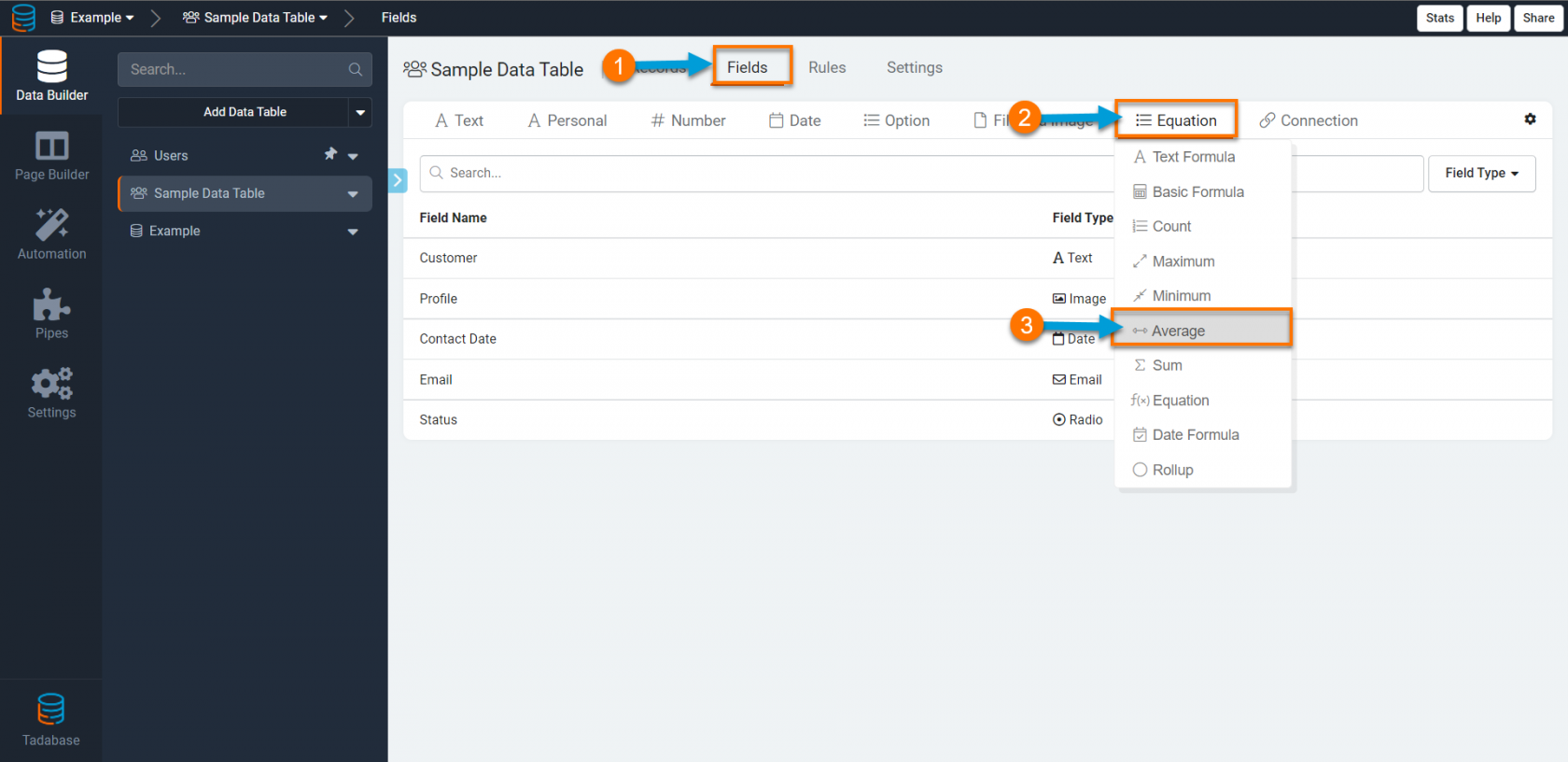When creating an Average field, there are several settings you can customize such as those highlighted in the image below.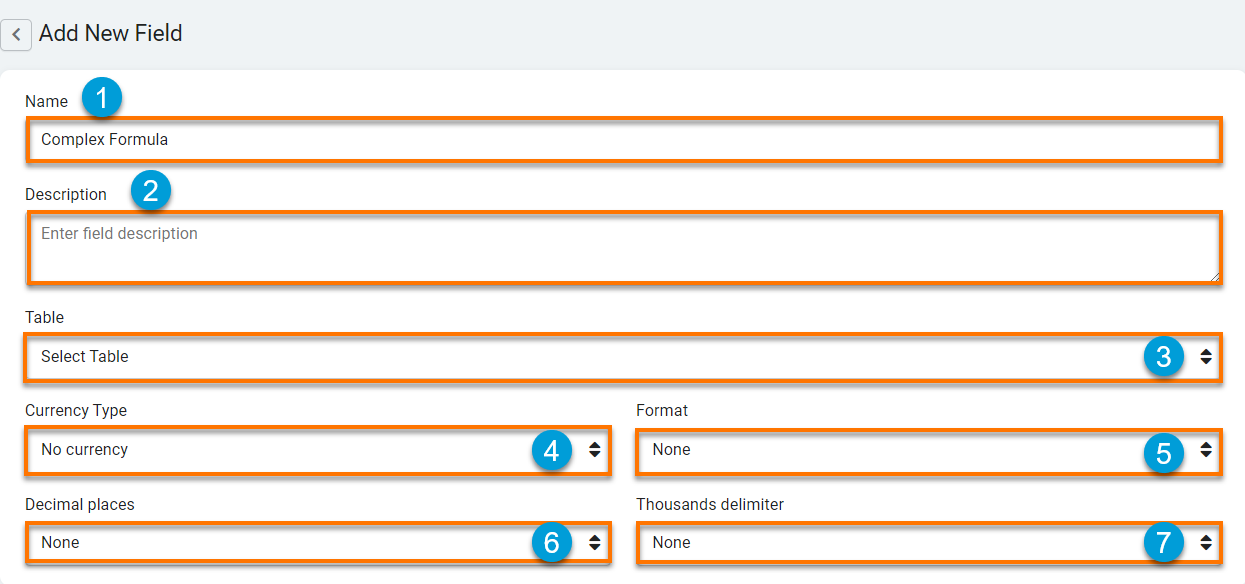1. Name- this is where you can name your Average field. For instance, "Average Grade" or "Average Cost".

2. Description- this is where you can optionally provide a brief description of this field for your own reference.

3. Table- this is where you can select the data table from which you would like to calculate the average value.

4. Currency Type- this is where you can choose a currency type to display within your Average field. At default, the currency type for average fields is set to No Currency. However, if you are creating an Average field to calculate a currency value you may wish to change this setting to a currency type of your choice, such as "\$- USD" or "€- EURO".

5. Format- this is where you can define the location of the currency symbol as it will display within your Average field values. At default, the currency symbol format for average fields is set to None, which means that no currency symbol will be displayed at default.

If desired, you can change the location formatting of the currency symbol to display either before or after your currency values. For instance, you can change the currency symbol formatting to Prefix which will display the currency symbol before your currency values as illustrated in the image below.6. Thousand Delimiter- this is where you can define which character you wish to use as a thousands delimiter to separate groups of thousands when viewing your currency values. At default, the thousands delimiter setting for average fields is set to None, meaning your currency values will display with no thousands delimiter at default and will display as such:If you wish to change your thousands delimiter setting and choose a delimiter character, you can choose either a commaperiod, or space character to separate groups of thousands within your currency values.

For instance, this is how your currency values will display if you change your thousands delimiter setting to Comma:7. Decimal Places- this is where you can define how many digits you wish to appear after the decimal within your average field values. At default, the decimal place setting for average fields is set to None, meaning average field values will display and be stored as whole digits at default.

#### An example: Creating an Average field to calculate each student's average grade.

For instance, say you have a Students data table, a Grades data table, and a connection field joining these two data tables which assigns each grade to a student. With an Average field, you can create a complex formula to calculate each student's Average Grade at any given time.

To create this average field, click on the Average field type on the Number field type category on the Add Field menu within the Students data table.Name your Average field "Average Grade" and configure your formula to calculate the average value from records within the Grades data table (where each grade is assigned to a student) using the Grade field. You can change the decimal places setting to display two digits after the decimal when displaying each student's Average Grade within components on the live app. Click Save to save your new Average Grade field.You have just added an Average Grade field to your Students data table that will calculate each student's average grade. You can view the results of your new average field in action as it would display on your live application via the image below.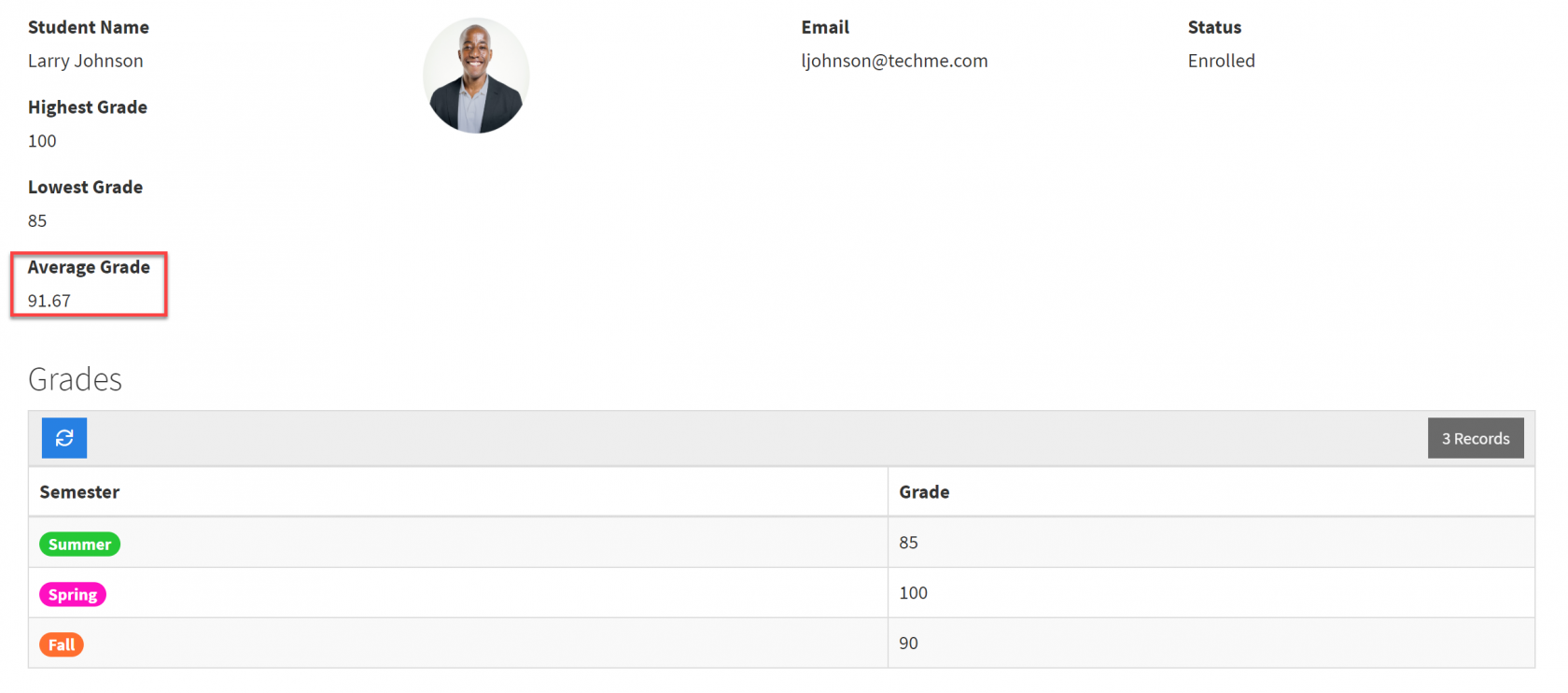## Sum

Sum fields are complex formula fields designed to sum records of a connected data table.

Unlike Basic Formulas, Complex Formulas such as Sum fields work with connected records.

For instance, say you have a Customers data table, a Jobs data table, and a connection field joining these two data tables which assigns each job to a customer. With a Sum field, you can create a complex formula to sum the Total Cost of each customer's jobs at any given time.

Sum fields can only be used to sum numeric fields.

Sample Sum Field: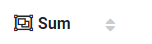### Creating a Sum Field

To add a new sum field to your data table, click on the Number field type category on the Add Field menu and select the sum field type from the drop-down list.When creating a Sum field, there are several settings you can customize such as those highlighted in the image below.1. Name- this is where you can name your sum field. For instance, "Total Cost" or "Total Expenses".

2. Description- this is where you can optionally provide a brief description of this field for your own reference.

3. Table- this is where you can select the data table from which you would like to sum records.

4. Currency Type- this is where you can choose a currency type to display within your sum field. At default, the currency type for sum fields is set to No Currency. However, if you are creating a sum field to calculate a currency value you may wish to change this setting to a currency type of your choice, such as "\$- USD" or "€- EURO".

5. Format-this is where you can define the location of the currency symbol as it will display within your sum field values. At default, the currency symbol format for sum fields is set to None, which means that no currency symbol will be displayed at default.

If desired, you can change the location formatting of the currency symbol to display either before or after your currency values. For instance, you can change the currency symbol formatting to Prefix which will display the currency symbol before your currency values as illustrated in the image below.6. Thousand Delimiter- this is where you can define which character you wish to use as a thousands delimiter to separate groups of thousands when viewing your currency values. At default, the thousands delimiter setting for sum fields is set to None, meaning your currency values will display with no thousands delimiter at default and will display as such:If you wish to change your thousands delimiter setting and choose a delimiter character, you can choose either a commaperiod, or space character to separate groups of thousands within your currency values.

For instance, this is how your currency values will display if you change your thousands delimiter setting to Comma:7. Decimal Places- this is where you can define how many digits you wish to appear after the decimal within your sum field values. At default, the decimal place setting for sum fields is set to None, meaning sum field values will display and be stored as whole digits at default.

#### An example: Creating a Sum field to sum the total cost of each customer's jobs.

For instance, say you have a Customers data table, a Jobs data table with a Costfield, and a connection field joining these two data tables which assigns each job to a customer. With a Sum field, you can create a complex formula to sum the Total Cost of each customer's jobs at any given time.

To create this sum field, click on the Sum field type on the Equation field type category on the Add Field menu within the Customers data table.Name your Sum field "Total Cost" and configure your formula to sum records from the Jobs data table (where each job is assigned to a customer) using the Cost field. Click Save to save your new Total Cost Sum field.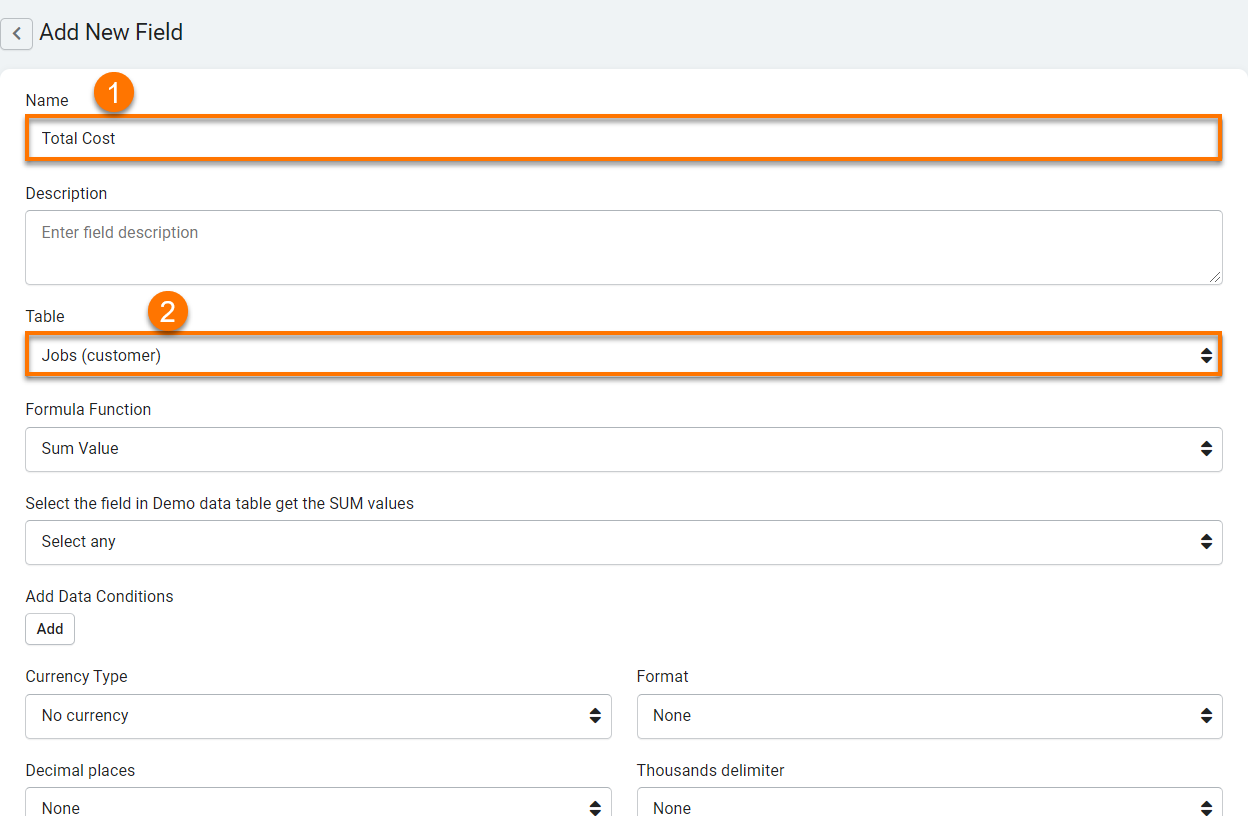You have just added a Total Cost Sum field to your Customers data table that will sum the total cost of each customer's jobs. You can view the results of your Sum field in action via the GIF below where you can see the Total Cost field for Customer John Simonds dynamically changing each time a new job is assigned to this customer.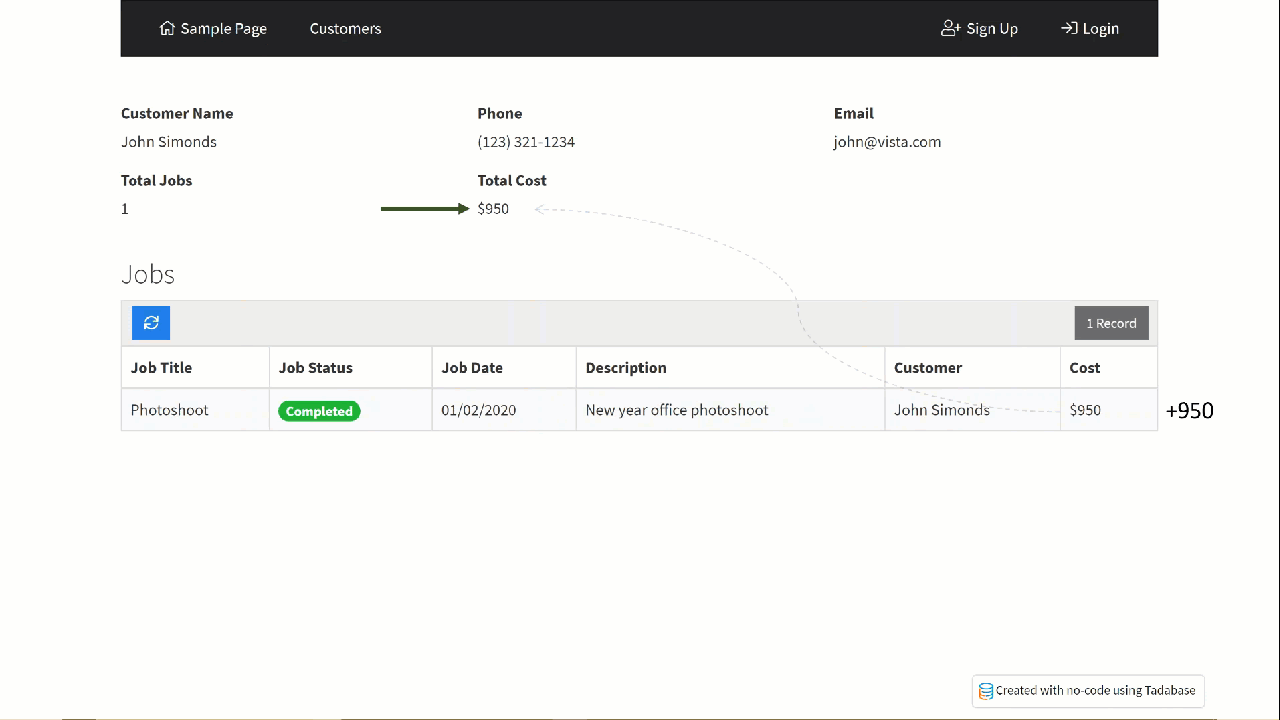As another example to demonstrate using sum complex formula fields, click here to learn how Sum fields are used in combination with IF equations to create automated reorder alerts for inventory tracking applications.

## Equations

Equation Fields enable more complex formula functions to be run on your values. As Tadabase offers a plethora of equation types and functions to utilize, we have created a separate article to discuss equations in entirety. Please continue to the following article to learn all about equations.

## Date Formula

Date Formula Fields contain automatically generated values based on a defined formula. A Date Formula field can reference other date field type values within its formula.

Sample Date Formula Field:### Creating a date formula field

To add a new date formula field to your data table, click on the Date field type category on the Add Field menu and select the date formula field type from the drop-down list.When creating a Date Formula field, there are several settings you can customize such as those highlighted in the image below.1. Name- this is where you can name your Date Formula field. For instance, "Total Time" or Total Hours".

2. Description- this is where you can optionally provide a brief description of this field for your own reference.

3. Display Output- this is where you can select the display output for your Date Formula field. For instance, if you are creating a date formula field to track the total hours of each employee's work day, you would choose Hour as the display output.

4. Date Formula- this is where you define your Date Formula by adding fields, operations, and custom text and date values.

For instance, say you have an Hours data table with a Clock In date/time field, a Clock Out date/time field, and a connection field to your employees. Your employees are clocking in and out every day and you would now like to track the Total Hours each employee is working each day. You can accomplish this by creating a Date Formula field titled Total Hours

To create this Date Formula field, click on the Equations category on the Add Field Menu within your Hours data table and select the Date Formula field type.Name your Date Formula "Total Hours" and select "Hour" as the Display Output with an hour format of "2.88 Hours" to display results as a numeric value representation of each employee's total hours worked each day (For instance, 8.75 Hours). You can then create your date formula by clicking on the +Add Field drop-down arrow and clicking on the Clock Out field to add this field to your formula. The reason why you are adding the Clock Out field first to your formula is because Total Hours = Clock Out (End Time) - Clock In (Start Time).As you can see, the Clock Out field has been added to your formula. You can then continue adding the remainder of your date formula by clicking on the - (minus) operator and then clicking on the +Add Field drop-down arrow once again to add the Clock In field. You can then click Save to save your new Total Hours date formula field.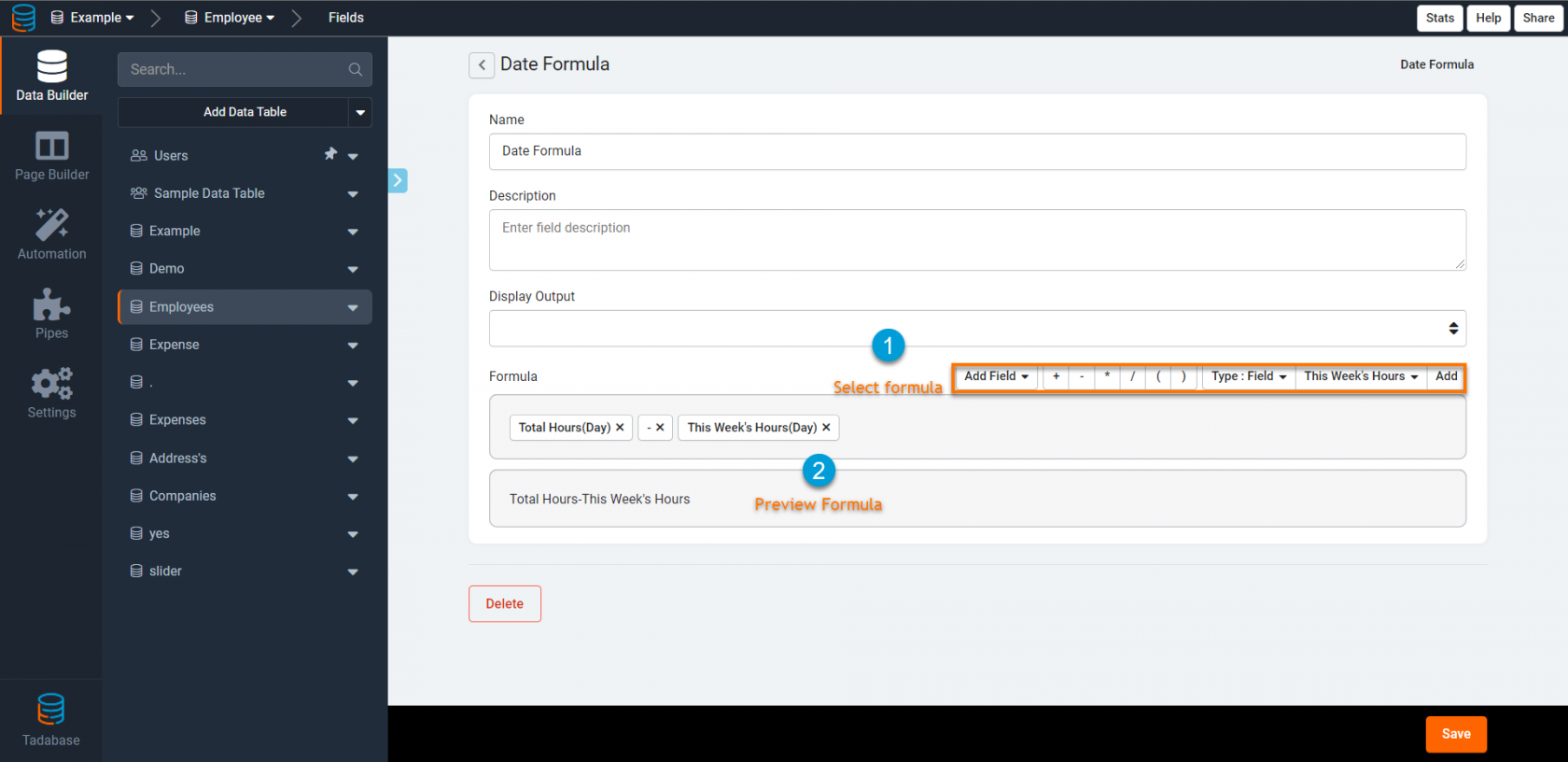The image below displays the results of your date formula field in action. As you can see, each employee's Clock In and Clock Out date/time fields are calculated into a new date formula field displaying his/her's total daily hours.